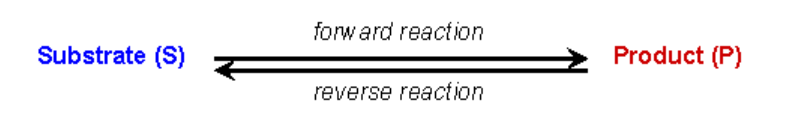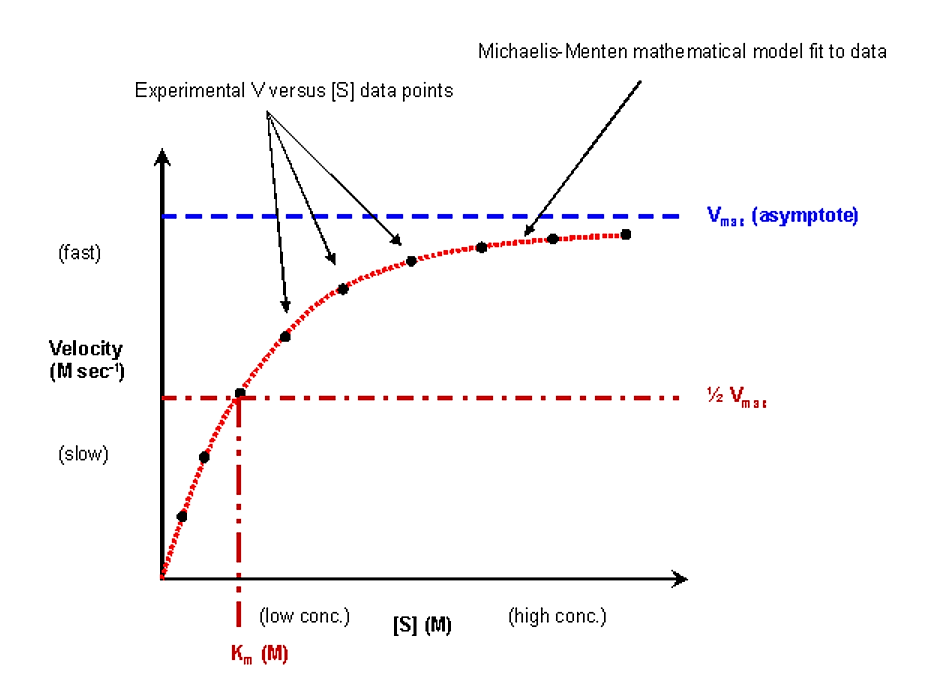# 6.2: Enzyme kinetics

$$\newcommand{\vecs}{\overset { \rightharpoonup} {\mathbf{#1}} }$$ $$\newcommand{\vecd}{\overset{-\!-\!\rightharpoonup}{\vphantom{a}\smash {#1}}}$$$$\newcommand{\id}{\mathrm{id}}$$ $$\newcommand{\Span}{\mathrm{span}}$$ $$\newcommand{\kernel}{\mathrm{null}\,}$$ $$\newcommand{\range}{\mathrm{range}\,}$$ $$\newcommand{\RealPart}{\mathrm{Re}}$$ $$\newcommand{\ImaginaryPart}{\mathrm{Im}}$$ $$\newcommand{\Argument}{\mathrm{Arg}}$$ $$\newcommand{\norm}{\| #1 \|}$$ $$\newcommand{\inner}{\langle #1, #2 \rangle}$$ $$\newcommand{\Span}{\mathrm{span}}$$ $$\newcommand{\id}{\mathrm{id}}$$ $$\newcommand{\Span}{\mathrm{span}}$$ $$\newcommand{\kernel}{\mathrm{null}\,}$$ $$\newcommand{\range}{\mathrm{range}\,}$$ $$\newcommand{\RealPart}{\mathrm{Re}}$$ $$\newcommand{\ImaginaryPart}{\mathrm{Im}}$$ $$\newcommand{\Argument}{\mathrm{Arg}}$$ $$\newcommand{\norm}{\| #1 \|}$$ $$\newcommand{\inner}{\langle #1, #2 \rangle}$$ $$\newcommand{\Span}{\mathrm{span}}$$$$\newcommand{\AA}{\unicode[.8,0]{x212B}}$$

Enzymes are protein catalysts, they influence the kinetics but not the thermodynamics of a reaction

• Increase the rate of a chemical reaction
• Do not alter the equilibriumFigure 6.2.1: Catalyst activity

• They increase the rate by stabilizing the transition state (i.e. lowering the energy barrier to forming the transition state (they do not affect the energetics of either the reactant(s) or product(s)

### Michaelis-Menten derivation for simple steady-state kinetics

The Michaelis-Menten equation is a mathematical model that is used to analyze simple kinetic data. The model has certain assumptions, and as long as these assumptions are correct, it will accurately model your experimental data. The derivation of the model will highlight these assumptions.In an enzyme catalyzed reaction the substrate initially forms a reversible complex with the enzyme (i.e. the enzyme and substrate have to interact for the enzyme to be able to perform its catalytic function). The standard expression to show this is the following:ASSUMPTION #1:

• There is no product present at the start of the kinetic analysis
• Therefore, as long as we monitor initial reaction rates we can ignore the reverse reaction of E+P going to ESASSUMPTION #2:

• During the reaction an equilibrium condition is established for the binding and dissociation of the Enzyme and Substrate (Briggs-Haldane assumption)
• Thus, the rate of formation of the ES complex is equal to the rate of dissociation plus breakdown

ASSUMPTION #3:

• [E] << [S]
• The enzyme is a catalyst, it is not destroyed and can be recycled, thus, only small amounts are required
• The amount of S bound to E at any given moment is small compared to the amount of free S
• It follows that [ES] << [S] and therefore [S] is constant during the course of the analysis (NOTE: this assumption requires that the reaction is monitored for a short period, so that not much S is consumed and [S] does not effectively change - see next assumption)

ASSUMPTION #4:

• Only the initial velocity of the reaction is measured
• [P] = 0 (reverse E + P reaction can be ignored)
• [S] » [S]initial

ASSUMPTION #5:

• The enzyme is either present as free enzyme or as the ES complex
• [E]total = [E] + [ES]

## Michaelis-Menten derivation using above assumptions:

Rate of ES formation = k1[E][S] + k-2[E][P]

Assumption #1 says we can ignore the k-2 reaction, therefore:

Rate of ES formation = k1[E][S]

Assumption #5 says [E] = [E]total - [ES], therefore:

Rate of ES formation = k1([E]total - [ES])[S]

The rate of ES breakdown is a combination of the dissociation and the conversion to product:

Rate of ES breakdown = k-1[ES] + k2[ES]

Rate of ES breakdown = (k-1 + k2)[ES]

Assumption #2 says the rate of ES formation equals the rate of breakdown:

k1([E]total - [ES])[S] = (k-1 + k2)[ES]

Rearrange to define in terms of rate constants:

([E]total - [ES])[S] / [ES] = (k-1 + k2) / k1

([E]total [S] / [ES]) - [S] = (k-1 + k2) / k1

Define a new constant, Km = (k-1 + k2) / k1

([E]total [S] / [ES]) - [S] = Km

Solve for the [ES] term (for reasons that will be given in the next step):

[ES] = [E]total [S] / (Km + [S])

The actual reaction velocity measured at any given moment is given by:

V = k2[ES]

Multiple both sides of the above equation by k2:

k2[ES] = k2[E]total [S] / (Km + [S])

thus

V = k2[E]total [S] / (Km + [S])

The maximum possible velocity (Vmax) occurs when all the enzyme molecules are bound with substrate [ES] = [E]total, thus:

Vmax = k2[E]total

Substituting this into the prior expression gives:

V = Vmax [S] / (Km + [S])

This is the mathematical expression that is used to model your experimental kinetic data

It is known as the Michaelis-Menten equation

## Experimental approach

The general approach is to add a known concentration of substrate to the enzyme and to determine the initial reaction rate for that concentration of substrate

• Reaction rates are typically given as moles (or micromole) of product produced per unit of time (sec or min) per mole (or micromole) of enzyme
• The experiment is repeated for a wide range of substrate concentrations
• A table of [S] versus V datapoints are collected
• These datapoints are plotted (V versus S) and should fit a curve that agrees with the Michaelis-Menten equation

The Vmax and Km terms are intrinsic properties of the particular enzyme/substrate combination that you are studying

• They will be determined from the features of the V versus S plot

Vmax

There are a limited number of enzyme molecules and they can only perform a single reaction at a time. Thus, at high [S] the enzymes can be saturated

• Under saturating conditions the reaction is going as fast as it can, and additional increases in [S] do not increase the reaction rate.
• The maximum observable rate is Vmax and the data will asymptote to this value at high [S]
• At low [S] the reaction rate is generally linearly proportional to the [S] (i.e. at low [S] if you double [S] the V will double)

Km

Km = (k-1 + k2) / k1 = (rate of breakdown of ES)/(rate of formation of ES)

• Km is similar, but not exactly equal to, a dissociation constant (Kd) for the ES complex
• If k-1 >> k2, then Km » Kd
• Due to this similarity to the expression for Kd, a low value of Km is often interpreted as a high affinity of the enzyme for the substrate, and a large value for Km is often interpreted as a weak affinity of the enzyme for the substrate
• Km has units of molar concentration (just like the units for [S])

There is a mathematical treatment that allows for the determination of Km from the experimental V versus [S] data

• Consider the situation when the [S] being evaluated results in a value of V that is exactly 1/2 of the maximum reaction velocity:

V = Vmax [S] / (Km + [S])

1/2Vmax = Vmax [S] / (Km + [S])

1/2 = [S] / (Km + [S])

Km + [S] = 2[S]

Km = [S]

Thus, Km equals the substrate concentration that results in exactly one half the maximum possible reaction velocityFigure 6.2.2: Km

## Lineweaver-Burke (the "double reciprocal" plot)

• The Michaelis-Menten equation can be rearranged by taking the reciprocal, to yield:• If X = 1/[S] and Y=1/V then this is a linear equation with a slope of Km/Vmax and a Y intercept of 1/VmaxFigure 6.2.3: 1/S and1/V

• Since the plot of 1/[S] versus 1/v data should be a straight line, it is easier to fit a linear function to the data in this form, and Vmax and Km can be readily determined from the plot

## Reversible Inhibition

There are two major categories of reversible inhibitors: competitive reversible inhibitors, and noncompetitive reversible inhibitors:

### Competitive inhibitors

The inhibitor (I) competes with the substrate (S) for the enzyme active site (also known as the S-binding site). Binding of either of these molecules in the active site is a mutually exclusive event

• The substrate and inhibitor share a high degree of structural similarity. However, the inhibitor cannot proceed through the reaction to produce product.
• Increasing the concentration of substrate will outcompete the inhibitor for binding to the enzyme active site
• A competitive reversible inhibitor can be identified by its characteristic effects upon kinetic data

The expression for the Michaelis-Menten expression in the presence of a reversible competitive inhibitor is:

V = Vmax [S] / (Km(1+[I]/Ki) + [S])

Where Ki is the actual EI complex dissociation constant

The effects of the reversible competitive inhibitor on the kinetics are as follows:

• If no inhibitor is present (i.e. if [I] = 0) then the equations are the same
• As inhibitor is added, the effect is to modify the apparent value of Km. In particular, the apparent Km will be increased by a value equal to (1 + [I]/KI). If Km is increased, the reaction velocity v will decrease.
• Note that as [S] gets very large the value of the denominator is essentially equal to [S] and v @ vmax. Thus, the reaction velocity can be driven to vmax with a high enough substrate concentration

The diagnostic criteria for reversible competitive inhibition is that while the apparent Km is affected by addition of the inhibitor, the value of vmax does not changeFigure 6.2.4: Effect of reversible competitive inhibitor

How is the Lineweaver-Burke double reciprocal plot affected by the presence of a reversible competitive inhibitor?Figure 6.2.5: Double reciprocal plot with reversible competitive inhibitor

### Noncompetitive Inhibitors

Noncompetitive inhibitors react with both E and ES (this is because the noncompetitive inhibitor does not bind at the same site in the enzyme as the substrate)

• Inhibition cannot be overcome by increasing the concentration of S
• The effect on kinetics is as if the enzyme were less active (vmax is reduced), but that the affinity for substrate is unaffected (Km remains the same) since the substrate binding site is not occupied by the noncompetitive inhibitor.Figure 6.2.6: Effect of reversible noncompetitive inhibitorFigure 6.2.7: Double reciprocal plot with noncompetitive inhibitor

This page titled 6.2: Enzyme kinetics is shared under a not declared license and was authored, remixed, and/or curated by Michael Blaber.